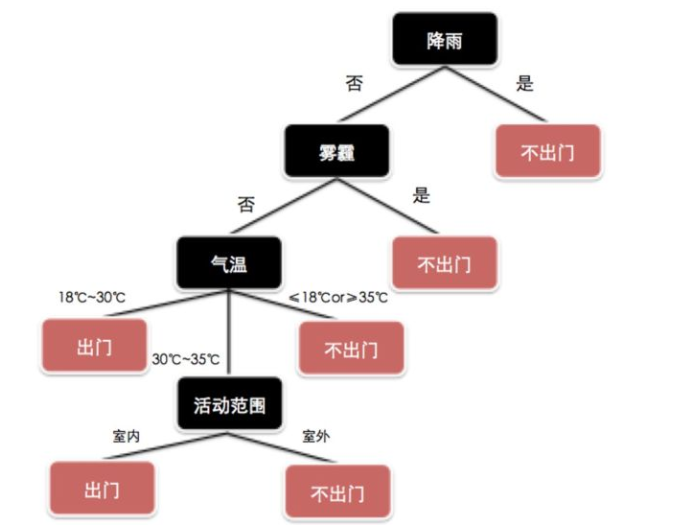# 决策树分类和回归入门实战和模型性能对比• 如何从数据表中找出最佳节点和最佳分枝？
决策树是对特征提问，如何找出最佳节点和最佳分支，我怎么知道哪些特征提问，才能生成有效的树呢？
• 如何让决策树停止增长，防止过拟合？
如果我有无数个特征，决策树会长成什么样子，他是不是会长成无数层深，我们要怎么样让它停止成长呢？怎么样防止过拟合呢？

### 1.1 决策树的优点

• 计算简单，易于理解，可解释性强;
• 比较适合处理有缺失属性的样本;
• 能够处理不相关的特征;
• 在相对短的时间内能够对大型数据源做出可行且效果良好的结果。

### 1.2 决策树的缺点

• 容易发生过拟合(随机森林可以很大程度上减少过拟合);
• 忽略了数据之间的相关性;
• 对于那些各类别样本数量不一致的数据，在决策树当中,信息增益的结果偏向于那些具有更多数值的特征(只要是>- 使用了信息增益，都有这个缺点，如RF)。

### 1.3 决策树参数

sklearn决策树的两个类：

tree.DecisionTreeClassifier()
tree.DecisionTreeRegressor()

Criterion这个参数正是用来决定不纯度的计算方法的。sklearn提供了两种选择：

• “entropy”，使用信息熵（Entropy）
• “gini”，使用基尼系数（Gini Impurity）

#### 决策树的重要参数random_state：

random_state用来设置分枝中的随机模式的参数，默认None，在高维度时随机性会表现更明显，低维度的数据（比如鸢尾花数据集），随机性几乎不会显现。输入任意整数，会一直长出同一棵树，让模型稳定下来。

#### 决策树的重要参数splitter：

splitter也是用来控制决策树中的随机选项的，有两种输入值，输入”best”，决策树在分枝时虽然随机，但是还是会优先选择更重要的特征进行分枝（重要性可以通过属性feature_importances_查看），输入“random”，决策树在分枝时会更加随机，树会因为含有更多的不必要信息而更深更大，并因这些不必要信息而降低对训练集的拟合。这也是防止过拟合的一种方式。当你预测到你的模型会过拟合，用这两个参数来帮助你降低树建成之后过拟合的可能性。当然，树一旦建成，我们依然是使用剪枝参数来防止过拟合。

#### 剪枝参数

##### 2. min_samples_leaf

min_samples_leaf 限定，一个节点在分枝后的每个子节点都必须包含至少min_samples_leaf个训练样本，否则分枝就不会发生，或者，分枝会朝着满足每个子节点都包含min_samples_leaf个样本的方向去发生

##### 3. min_samples_split

min_samples_split限定，一个节点必须要包含至少min_samples_split个训练样本，这个节点才允许被分枝，否则分枝就不会发生。

### 2.1 准备数据

iris = pd.read_csv('http://archive.ics.uci.edu/ml/machine-learning-databases/iris/iris.data',header=None)
iris.columns=['SepalLengthCm','SepalWidthCm','PetalLengthCm','PetalWidthCm','Species']

le = LabelEncoder()
le.fit(iris['Species'])
features =  ['SepalWidthCm','PetalWidthCm']
X = iris[features]
y = le.transform(iris['Species'])

### 2.2 进行分类

tr = tree.DecisionTreeClassifier()
tr.fit(X,y)
score = numpy.mean(cross_val_score(tr,X,y,cv=5,scoring='accuracy'))
print('决策树分类模型平均性能得分：'+str(score))

tr = tree.DecisionTreeClassifier(criterion="entropy",random_state=10,splitter="best",max_depth=10,min_samples_leaf=5,min_samples_split=5)
score = numpy.mean(cross_val_score(tr,X,y,cv=5,scoring='accuracy'))
print('决策树分类模型平均性能得分：'+str(score))

### 2.3 和logistic分类对比

lm = linear_model.LogisticRegression()
score = numpy.mean(cross_val_score(lm,X,y,cv=5,scoring='accuracy'))
print('logistic回归模型平均性能得分：'+str(score))

### 3.1 进行回归

y = iris['PetalWidthCm']
tr = tree.DecisionTreeRegressor()
score = numpy.mean(-cross_val_score(tr,X,y,cv=5,scoring='neg_mean_squared_error'))
print('平均性能得分：'+str(score))

### 3.2 和线性回归对比

lm = linear_model.LinearRegression()
score = numpy.mean(-cross_val_score(tr,X,y,cv=5,scoring='neg_mean_squared_error'))
print('线性回归模型平均性能得分：'+str(score))

import pandas as pd
from sklearn.model_selection import cross_val_score
import numpy
from sklearn.preprocessing import LabelEncoder
from sklearn import linear_model
from sklearn import tree
from sklearn import ensemble

iris.columns=['SepalLengthCm','SepalWidthCm','PetalLengthCm','PetalWidthCm','Species']

le = LabelEncoder()
le.fit(iris['Species'])
features =  ['SepalWidthCm','PetalWidthCm']
X = iris[features]
y = le.transform(iris['Species'])

tr = tree.DecisionTreeClassifier(criterion="entropy",random_state=10,splitter="best",max_depth=10,min_samples_leaf=5,min_samples_split=5)
score = numpy.mean(cross_val_score(tr,X,y,cv=5,scoring='accuracy'))
print('决策树分类模型平均性能得分：'+str(score))

lm = linear_model.LogisticRegression()
score = numpy.mean(cross_val_score(lm,X,y,cv=5,scoring='accuracy'))
print('logistic回归模型平均性能得分：'+str(score))

y = iris['PetalWidthCm']
tr = tree.DecisionTreeRegressor()
score = numpy.mean(-cross_val_score(tr,X,y,cv=5,scoring='neg_mean_squared_error'))
print('决策树回归模型平均性能得分：'+str(score))

lm = linear_model.LinearRegression()
score = numpy.mean(-cross_val_score(tr,X,y,cv=5,scoring='neg_mean_squared_error'))
print('线性回归模型平均性能得分：'+str(score))

#### 请关注我的微信公众号